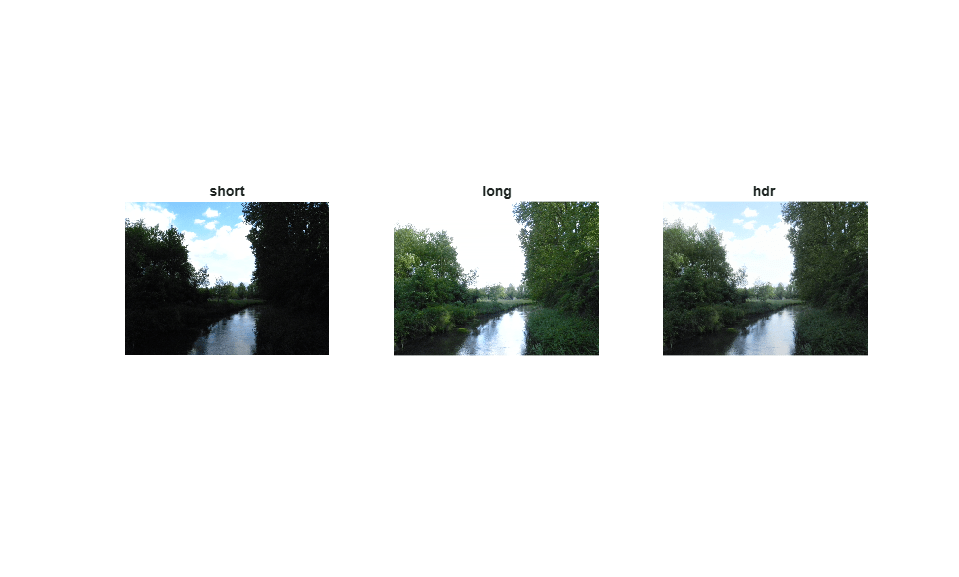# High Dynamic Range Imaging

This example shows how to generate HDL code from a MATLAB® design that implements a high dynamic range imaging algorithm.

### Algorithm

High Dynamic Range Imaging (HDRI or HDR) is a set of methods used in imaging and photography to allow a greater dynamic range between the lightest and darkest areas of an image than current standard digital imaging methods or photographic methods. HDR images can represent more accurately the range of intensity levels found in real scenes, from direct sunlight to faint starlight, and is often captured by way of a plurality of differently exposed pictures of the same subject matter.

### MATLAB Design

```design_name = 'mlhdlc_hdr'; testbench_name = 'mlhdlc_hdr_tb'; ```

Use the `dbtype` function to display the contents of the MATLAB design.

```dbtype(design_name); ```
```1 function [valid_out, x_out, y_out, ... 2 HDR1, HDR2, HDR3] = mlhdlc_hdr(YShort1, YShort2, YShort3, ... 3 YLong1, YLong2, YLong3, ... 4 plot_y_short_in, plot_y_long_in, ... 5 valid_in, x, y) 6 % 7 8 % Copyright 2013-2015 The MathWorks, Inc. 9 10 % This design implements a high dynamic range imaging algorithm. 11 12 plot_y_short = plot_y_short_in; 13 plot_y_long = plot_y_long_in; 14 15 %% Apply Lum(Y) channels LUTs 16 y_short = plot_y_short(uint8(YShort1)+1); 17 y_long = plot_y_long(uint8(YLong1)+1); 18 19 y_HDR = (y_short+y_long); 20 21 %% Create HDR Chorm channels 22 % HDR per color 23 24 HDR1 = y_HDR * 2^-8; 25 HDR2 = (YShort2+YLong2) * 2^-1; 26 HDR3 = (YShort3+YLong3) * 2^-1; 27 28 %% Pass on valid signal and pixel location 29 30 valid_out = valid_in; 31 x_out = x; 32 y_out = y; 33 34 end ```
```dbtype(testbench_name); ```
```1 2 % 3 4 % Copyright 2013-2015 The MathWorks, Inc. 5 6 % Clean screen and memory 7 close all 8 clear mlhdlc_hdr 9 set(0,'DefaultFigureWindowStyle','docked') 10 11 12 %% Read the two exposed images 13 14 short = imread('mlhdlc_hdr_short.tif'); 15 long = imread('mlhdlc_hdr_long.tif'); 16 17 % define HDR output variable 18 HDR = zeros(size(short)); 19 [height, width, color] = size(HDR); 20 21 set(0,'DefaultFigureWindowStyle' , 'normal') 22 figure('Name', [mfilename, '_plot']); 23 subplot(1,3,1); 24 imshow(short, 'InitialMagnification','fit'), title('short'); 25 26 subplot(1,3,2); 27 imshow(long, 'InitialMagnification','fit'), title('long'); 28 29 30 %% Create the Lum(Y) channels LUTs 31 % Pre-process 32 % Luminance short LUT 33 ShortLut.x = [0 16 45 96 255]; 34 ShortLut.y = [0 20 38 58 115]; 35 36 % Luminance long LUT 37 LongLut.x = [ 0 255]; 38 LongLut.y = [ 0 140]; 39 40 % Take the same points to plot the joined Lum LUT 41 plot_x = 0:1:255; 42 plot_y_short = interp1(ShortLut.x,ShortLut.y,plot_x); %LUT short 43 plot_y_long = interp1(LongLut.x,LongLut.y,plot_x); %LUT long 44 45 %subplot(4,1,3); 46 %plot(plot_x, plot_y_short, plot_x, plot_y_long, plot_x, (plot_y_long+plot_y_short)), grid on; 47 48 49 %% Create the HDR Lum channel 50 % The HDR algorithm 51 % read the Y channels 52 53 YIQ_short = rgb2ntsc(short); 54 YIQ_long = rgb2ntsc(long); 55 56 %% Stream image through HDR algorithm 57 58 for x=1:width 59 for y=1:height 60 YShort1 = round(YIQ_short(y,x,1)*255); %input short 61 YLong1 = round(YIQ_long(y,x,1)*255); %input long 62 63 YShort2 = YIQ_short(y,x,2); %input short 64 YLong2 = YIQ_long(y,x,2); %input long 65 66 YShort3 = YIQ_short(y,x,3); %input short 67 YLong3 = YIQ_long(y,x,3); %input long 68 69 valid_in = 1; 70 71 [valid_out, x_out, y_out, HDR1, HDR2, HDR3] = mlhdlc_hdr(YShort1, YShort2, YShort3, YLong1, YLong2, YLong3, plot_y_short, plot_y_long, valid_in, x, y); 72 73 % use x and y to reconstruct image 74 if valid_out == 1 75 HDR(y_out,x_out,1) = HDR1; 76 HDR(y_out,x_out,2) = HDR2; 77 HDR(y_out,x_out,3) = HDR3; 78 end 79 end 80 end 81 82 %% plot HDR 83 HDR_rgb = ntsc2rgb(HDR); 84 subplot(1,3,3); 85 imshow(HDR_rgb, 'InitialMagnification','fit'), title('hdr '); ```

### Simulate the Design

It is always a good practice to simulate the design with the testbench prior to code generation to make sure there are no runtime errors.

```mlhdlc_hdr_tb ```### Create a New HDL Coder™ Project

```coder -hdlcoder -new mlhdlc_hdr_prj ```

Next, add the file 'mlhdlc_hdr.m' to the project as the MATLAB Function and 'mlhdlc_hdr_tb.m' as the MATLAB Test Bench.

Refer to Get Started with MATLAB to HDL Workflow for a more complete tutorial on creating and populating MATLAB HDL Coder projects.

### Creating Constant Parameter Inputs

This example shows to use pass constant parameter inputs.

In this design the input parameters 'plot_y_short_in' and 'plot_y_long_in' are constant input parameters. You can define them accordingly by modifying the input types as 'constant(double(1x256))'

'plot_y_short_in' and 'plot_y_short_in' are LUT inputs. They are constant folded as double inputs to the design. You will not see port declarations for these two input parameters in the generated HDL code.

Note that inside the design 'mlhdlc_hdr.m' these variables are reassigned so that they get properly fixed-point converted. This is not necessary if these are purely used as constants for defining sizes of variables for example and not part of the logic.

### Run Fixed-Point Conversion and HDL Code Generation

Launch HDL Advisor and right click on the 'Code Generation' step and choose the option 'Run to selected task' to run all the steps from the beginning through the HDL code generation.

### Convert the Design to Fixed-Point and Generate HDL Code

The following script converts the design to fixed-point, and generate HDL code with a test bench.

``` exArgs = {0,0,0,0,0,0,coder.Constant(ones(1,256)),coder.Constant(ones(1,256)),0,0,0}; fc = coder.config('fixpt'); fc.TestBenchName = 'mlhdlc_hdr_tb'; hc = coder.config('hdl'); hc.GenerateHDLTestBench = true; hc.SimulationIterationLimit = 1000; % Limit number of testbench points codegen -float2fixed fc -config hc -args exArgs mlhdlc_hdr```

Examine the generated HDL code by clicking on the hyperlinks in the Code Generation Log window.